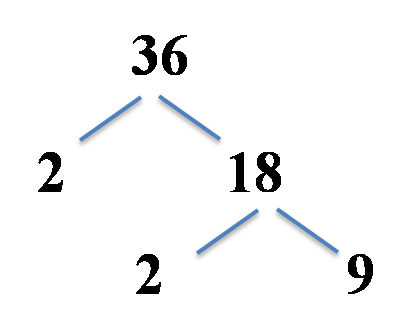d Factorise 9x²-81 | Job Exam Rare Mathematics (JEMrare) - Solved MCQs

# Question: Factorise 9x²-81

This question can be solved by using a^2-b^2 formula

$9x^2-81=(3x)^2-(9)^2$

$=(3x-9)(3x+9)$

$=9(x-3)(x+3)$

So, this is the factorisation of the term.

a^2-b^2 formula is to be used for this question.. Lastly common factor is to be taken out. Also we can such type Factorise 9x²-81 or x^2-64 etc ,

So, such questions seem difficult but are easy to tackleCopyright secured by Digiprove © 2021